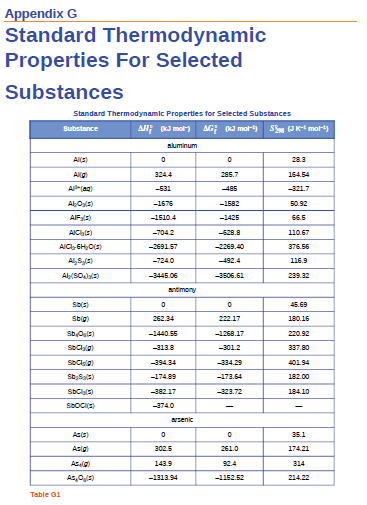# Problem: Using the relevant S°298 values listed in Appendix G, calculate ΔS°298 for the following change:(a) N2(g) + 3H2(g) ⟶ 2NH3(g)

83% (36 ratings)
###### Problem Details

Using the relevant S°298 values listed in Appendix G, calculate ΔS°298 for the following change:

(a) N2(g) + 3H2(g) ⟶ 2NH3(g)